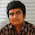## Welcome to the World of Modelling and Simulation

### What is Modelling?

This blog is all about system dynamics modelling and simulation applied in the engineering field, especially mechanical, electrical, and ...### Fourth Order Runge Kutta Method by MATLAB to Solve System of Differential Equations

The fourth-order Runge-Kutta method (RK4) is a widely used numerical approach to solve the system of differential equations. In this module, we will solve a system of three ordinary differential equations by implementing the RK4 algorithm in MATLAB.

x' = -5x + 5y

y' = 14x - 2y - zx

z' = -3z + xy

The initial conditions are, x(0) = y(0) = z(0) = 1

The following MATLAB program implements RK4 scheme to solve the above system of three differential equations.

close all;

clc;
format long;

% Defining the three differential equations of the problem
f=@(t,y) [-5*y(1)+5*y(2), 14*y(1)-2*y(2)-y(1)*y(3), -3*y(3)+y(1)*y(2)];
[x,y]=runge_kutta_4(f,[0,5],[1,1,1],0.1); % Calling RK4 function defined below
plot(x,y);
title('When Time Step is 0.1');
legend('x(t)', 'y(t)', 'z(t)', 'Location', 'NorthEast')
xlabel('t')
ylabel('Solutions')
figure;
hold on;

for h=[0.1, 10^-3, 10^-6] % Implementing 3 different time steps
[x,y]=runge_kutta_4(f,[0,5],[1,1,1],h);
plot(x,y(:,1));
end

title('Plot of x(t) for 3 Different Time Steps');
legend('h=0.1', 'h=10^{-3}', 'h=10^{-6}');
xlabel('t')
ylabel('x(t)')

% Fourth order Runge-Kutta method
function [x,y]=runge_kutta_4(f,tspan,y0,h)
x = tspan(1):h:tspan(2); % Calculates upto y(3)
y = zeros(length(x),3);
y(1,:) = y0; % Initial Conditions
for i=1:(length(x)-1)
k_1 = f(x(i),y(i,:));
k_2 = f(x(i)+0.5*h,y(i,:)+0.5*h*k_1);
k_3 = f((x(i)+0.5*h),(y(i,:)+0.5*h*k_2));
k_4 = f((x(i)+h),(y(i,:)+k_3*h));
y(i+1,:) = y(i,:) + (1/6)*(k_1+2*k_2+2*k_3+k_4)*h;
end

end

Program Output:

1.Thank you, these codes are helpful.

1.2.y'(t)=3e^-t -0.4y 0<t<2,y(0)=5
plz solved it

1.Hi there, you may use the codes shown in the post. In your case, it is more straightforward since you have one equation. You just need to define your equation in the following form:

% Defining the three differential equations of the problem
f=@(t,y) [-5*y(1)+5*y(2), 14*y(1)-2*y(2)-y(1)*y(3), -3*y(3)+y(1)*y(2)];

3.how can i use this code to solve ODEs of 5 variable

1.Hi Alok, you may apply the same method for 5 variables as well, you just need to arrange the equations in the same format shown in the post, and you have available initial conditions.

4.Thanks for sharing the code, but I got this result (Unrecognized function or variable 'runge_kutta_4'.
). Could you please tell what is wrong?

5.i need to solve with y(4) what can i do ?

6.7.I have a set differential equation with four equations that I solved through Rong-kotta of the fourth order, but it does not give correct answers. Where do you think the code is wrong?

% Initialise derivative functions
dy1 = @(t, y1, y2, y3, y4) y2; % dy1 = y1' = dy1/dt
dy2 = @(t, y1, y2, y3, y4) -(1./(MM1)).* ( C1.*y2 + K11.*y1 + K21.*y3 + K31.*y1.*y3 +...
K41.*y1.*y1 + K51.* y1.*y1.*y1 + F1 ); % dy2 = y2' = dy2/dt
dy3 = @(t, y1, y2, y3, y4) y4; % dy3 = y3' = dy3/dt
dy4 = @(t, y1, y2, y3, y4) -(1./MM2).* ( C2.*y4 + K12.*y3 + K22.*y1 + K32.*y1.*y1 );%dy4 = y4' = dy4/dt
%%
% Initialise K vectors
ky1 = zeros(1,4); % to store K values for y1
ky2 = zeros(1,4); % to store K values for y2
ky3 = zeros(1,4); % to store K values for y3
ky4 = zeros(1,4); % to store K values for y4
b = [1 2 2 1]; % RK4 coefficients
%%

% Iterate, computing each K value in turn, then the i+1 step values
for i = 1:(N-1)
ky1(1) = dy1(t(i), y1(i), y2(i), y3(i), y4(i));
ky2(1) = dy2(t(i), y1(i), y2(i), y3(i), y4(i));
ky3(1) = dy3(t(i), y1(i), y2(i), y3(i), y4(i));
ky4(1) = dy4(t(i), y1(i), y2(i), y3(i), y4(i));

ky1(2) = dy1(t(i) + (h/2), y1(i) + (h/2)*ky1(1), y2(i) + (h/2)*ky2(1), y3(i) + (h/2)*ky3(1), y4(i) + (h/2)*ky4(1));
ky2(2) = dy2(t(i) + (h/2), y1(i) + (h/2)*ky1(1), y2(i) + (h/2)*ky2(1), y3(i) + (h/2)*ky3(1), y4(i) + (h/2)*ky4(1));
ky3(2) = dy3(t(i) + (h/2), y1(i) + (h/2)*ky1(1), y2(i) + (h/2)*ky2(1), y3(i) + (h/2)*ky3(1), y4(i) + (h/2)*ky4(1));
ky4(2) = dy4(t(i) + (h/2), y1(i) + (h/2)*ky1(1), y2(i) + (h/2)*ky2(1), y3(i) + (h/2)*ky3(1), y4(i) + (h/2)*ky4(1));

ky1(3) = dy1(t(i) + (h/2), y1(i) + (h/2)*ky1(2), y2(i) + (h/2)*ky2(2), y3(i) + (h/2)*ky3(2), y4(i) + (h/2)*ky4(2));
ky2(3) = dy2(t(i) + (h/2), y1(i) + (h/2)*ky1(2), y2(i) + (h/2)*ky2(2), y3(i) + (h/2)*ky3(2), y4(i) + (h/2)*ky4(2));
ky3(3) = dy3(t(i) + (h/2), y1(i) + (h/2)*ky1(2), y2(i) + (h/2)*ky2(2), y3(i) + (h/2)*ky3(2), y4(i) + (h/2)*ky4(2));
ky4(3) = dy4(t(i) + (h/2), y1(i) + (h/2)*ky1(2), y2(i) + (h/2)*ky2(2), y3(i) + (h/2)*ky3(2), y4(i) + (h/2)*ky4(2));

ky1(4) = dy1(t(i) + h, y1(i) + h*ky1(3), y2(i) + h*ky2(3), y3(i) + h*ky3(3), y4(i) + h*ky4(3));
ky2(4) = dy2(t(i) + h, y1(i) + h*ky1(3), y2(i) + h*ky2(3), y3(i) + h*ky3(3), y4(i) + h*ky4(3));
ky3(4) = dy3(t(i) + h, y1(i) + h*ky1(3), y2(i) + h*ky2(3), y3(i) + h*ky3(3), y4(i) + h*ky4(3));
ky4(4) = dy4(t(i) + h, y1(i) + h*ky1(3), y2(i) + h*ky2(3), y3(i) + h*ky3(3), y4(i) + h*ky4(3));

y1(i+1) = y1(i) + (h/6)*sum(b.*ky1);
y2(i+1) = y2(i) + (h/6)*sum(b.*ky2);
y3(i+1) = y3(i) + (h/6)*sum(b.*ky3);
y4(i+1) = y4(i) + (h/6)*sum(b.*ky3);

end

% Group together in one solution matrix
% ty1y2y3y4 = [t,y1,y2,y3,y4]
% plot(t,y1)

figure(3)
plot(t,y1)
xlabel('t (sec)')
ylabel('y1')
grid on
hold on
%%
% subplot(3,1,2)
figure(4)
plot(t,y2)
xlabel('t')
ylabel('y2')
grid on
%%
% subplot(3,1,3)
figure(5)
plot(y1,y2) %% nemodar safahaat fazi
xlabel('y1')
ylabel('y2')
grid on
%%
figure(6)
plot(t,y3)
xlabel('t (sec)')
% ylabel('x1(Non-dimensional center gap)')
ylabel('y3')
grid on
hold on
%%
figure (7)
plot(t,y4,'r')
xlabel('t')
ylabel('y4')
grid on
%%
figure(8)
plot(y3,y4,'r') %% nemodar safahaat fazi
xlabel('y3')
ylabel('y4')
grid on

1.I will check your program later and let you know. Thanks!